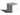## wayne的投影面积

Tue, 28th January 2020Edit on Github几何极值

2013年7月wayne提问:

# 具体内容

gxqcn认为对于第一个问题： 可以考虑从正方形中心向某个顶点引一条射线， 然后将该顶点绕中心逆时针从0°旋转到45°， 在旋转过程中，该顶点在射线上的投影与射线顶点间的线段长度即是正方形在该射线所在直线上投影长度的一半。

KeyTo9_Fans得出: 假设正方形的边长是$1$， 那么问题$1$的期望值是： $\frac{\sqrt{2}\int_0^{\frac{\pi}4}\cos(x)dx}{\frac{\pi}4}$ $=\frac{\sqrt{2}\sin(\frac{\pi}4)}{\frac{\pi}4}$ $=\frac{4}{\pi}$.

hujunhua指出这一点可以从球体直接得证，不需要进行积分计算。在这个面积元的中心法线上任取一点作为定点，这个面积元在绕此定点转遍所有的空间方向后刚好构成一个球面。

Github